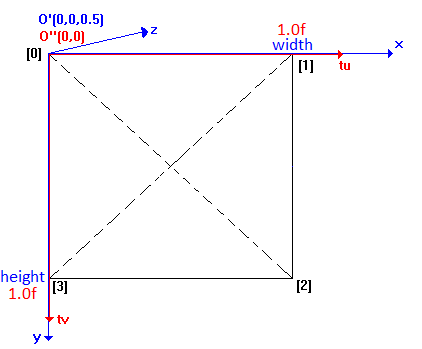# VDJPedia

Plugins_SDKv8_VideoExplanations

Video Effects & Transitions in VirtualDJ 8 (How To in Direct3D 9)

1) The first step in order to use Direct3D9 is to have to get a pointer to IDirect3DDevice9 that is defined by the VirtualDJ sofware. This pointer is available in the VirtualDJ video plugins SDK by using the following function:

`IDirect3DDevice9 *D3DDevice;void *device = NULL;HRESULT hr;hr = GetDevice(VdjVideoEngineDirectX9, &device);if(hr!=S_OK || device==NULL) return S_FALSE;D3DDevice = reinterpret_cast<IDirect3DDevice9*>(device);`

Each time you want to use a Direct3D functions, just use:

`D3DDevice->xxxxxxxxxxx`

2) A vertex is a dot in the space defined by the following structure
(NB: one vertex, two vertices)

`struct TVertex{	struct {FLOAT x,y,z;} position; // The 3D position (coordinates) for the vertex	DWORD color; // The vertex color	FLOAT tu,tv; // The texture coordinates};`

3D models in Direct3D are based on triangles:
To define a triangle, you need 3 Vertices: vertices, vertices, vertices
To define a square, you need 4 Vertices: vertices, vertices, vertices, vertices and in other words you need 2 triangles : {vertices, vertices, vertices} and {vertices, vertices, vertices}

SDK 8 | SDK 7 (and previous):It returns the following data fo the pointer Vertex:
(width and height are available in the VirtualDJ video plugins SDK)
(For information: D3DCOLOR_RGBA(255,255,255,255) = 4294967295)
`Vertex.position.x=0.0f * (float) width;	Vertex.position.y=0.0f * (float) height;	Vertex.position.z=0.5f;	Vertex.color=D3DCOLOR_RGBA(255,255,255,255);	Vertex.tu=0.0f;	Vertex.tv=0.0f;	Vertex.position.x= 1.0f * (float) width;	Vertex.position.y=0.0f * (float) height;	Vertex.position.z=0.5f;	Vertex.color=D3DCOLOR_RGBA(255,255,255,255);	Vertex.tu=1.0f;	Vertex.tv=0.0f;	Vertex.position.x=1.0f * (float) width;	Vertex.position.y=1.0f * (float) height;	Vertex.position.z=0.5f;	Vertex.color=D3DCOLOR_RGBA(255,255,255,255);	Vertex.tu=1.0f;	Vertex.tv=1.0f;	Vertex.position.x=0.0f * (float) width;;	Vertex.position.y=1.0f * (float) height;	Vertex.position.z=0.5f;	Vertex.color=D3DCOLOR_RGBA(255,255,255,255);	Vertex.tu=0.0f;	Vertex.tv=1.0f;`

3) Texturing:

"IDirect3DTexture9 *texture" is the current video texture

Then you apply the texture (video,bitmap,...) on this square with the following function:

D3DDevice->SetTexture(0,texture);

NB: in this case "0" means the first stage of the video

For a Video FX:
`IDirect3DTexture9 *texture;TVertex *Vertex;HRESULT hr;hr = GetTexture(VdjVideoEngineDirectX9, (void **) &texture, &Vertex);`

4) Renderering:

return S_FALSE; will automatically render the video for you

if you want to render by yourself, you can use for example the following function:
D3DDevice->DrawPrimitiveUP(D3DPT_TRIANGLESTRIP,2,(LPVOID)Vertices,sizeof(Vertices));
and in this case, use return S_OK;

Some source code examples:

`#ifndef SAFE_RELEASE	#define SAFE_RELEASE(x) { if (x!=NULL) { x->Release(); x=NULL; } }#endifHRESULT VDJ_API CMyPlugin8::OnLoad(){D3DDevice = NULL;g_font = NULL;return S_OK;}HRESULT VDJ_API CMyPlugin8::OnDeviceInit(){GetDevice(VdjVideoEngineDirectX9, (void **)&D3DDevice);D3DXCreateFont(D3DDevice, 22, 0, FW_NORMAL, 1, false, DEFAULT_CHARSET, OUT_DEFAULT_PRECIS, ANTIALIASED_QUALITY, DEFAULT_PITCH | FF_DONTCARE, "Arial", &g_font);return S_OK;}HRESULT VDJ_API CMyPlugin8::OnDraw(){if (!g_font || !D3DDevice) return S_FALSE;DrawDeck();RECT font_rect;SetRect(&font_rect, 0, 0, width, height);g_font->DrawText(NULL, fps_string, -1, &font_rect, DT_LEFT | DT_NOCLIP, 0xFFFFFFFF);return S_OK;}HRESULT VDJ_API CMyPlugin8::OnDeviceClose(){SAFE_RELEASE(g_font);D3DDevice = NULL;return S_OK;}`

If you're wanting to draw the default VDJ video with modified vertices, then do something like this:

`TVertex *v;IDirect3DTexture9 *t;GetTexture(VdjVideoEngineAny, (void**)&t, &v);// modify vertices hereDrawDeck();`

Finally if you're wanting to do something with your own vertices then have a play with something like this:

`#define D3DFVF_TLVERTEX3D (D3DFVF_XYZ|D3DFVF_DIFFUSE|D3DFVF_TEX1)HRESULT VDJ_API CMyPlugin8::OnDeviceInit(){	GetDevice(VdjVideoEngineAny, (void**)&device);	if (FAILED(device->CreateVertexBuffer(4 * sizeof(TVertex), D3DUSAGE_WRITEONLY, D3DFVF_TLVERTEX3D, D3DPOOL_DEFAULT, &vertexBuffer, NULL)))		return E_FAIL;	return S_OK;}HRESULT VDJ_API CMyPlugin8::OnDeviceClose(){	SAFE_RELEASE(vertexBuffer);	device = NULL;	return S_OK;}bool CMyPlugin8::UpdateVertices(){	if (FAILED(vertexBuffer->Lock(0, 0, (void**)&vertices, 0)))	{		vertexBuffer->Release();		vertexBuffer = NULL;		return false;	}	vertices.position.x = 0.0f;	vertices.position.y = 0.0f;	vertices.position.z = 0.5f;	vertices.color = D3DCOLOR_RGBA(255, 255, 255, 255);	vertices.tu = 0.f;	vertices.tv = 0.f;	vertices.position.x = (float)width;	vertices.position.y = 0.0f;	vertices.position.z = 0.5f;	vertices.color = D3DCOLOR_RGBA(255, 255, 255, 255);	vertices.tu = 1.f;	vertices.tv = 0.f;	vertices.position.x = (float)width;	vertices.position.y = (float)height;	vertices.position.z = 0.5f;	vertices.color = D3DCOLOR_RGBA(255, 255, 255, 255);	vertices.tu = 1.f;	vertices.tv = 1.f;	vertices.position.x = 0.0f;	vertices.position.y = (float)height;	vertices.position.z = 0.5f;	vertices.color = D3DCOLOR_RGBA(255, 255, 255, 255);	vertices.tu = 0.f;	vertices.tv = 1.f;	vertexBuffer->Unlock();	return true;}HRESULT VDJ_API CMyPlugin8::OnDraw(){	if (!device)		return S_FALSE;	TVertex *v;	IDirect3DTexture9 *t;	GetTexture(VdjVideoEngineAny, (void**)&t, &v);	UpdateVertices();	device->SetTexture(0, t);	device->SetStreamSource(0, vertexBuffer, 0, sizeof(TVertex));	device->SetFVF(D3DFVF_TLVERTEX3D);	device->DrawPrimitiveUP(D3DPT_TRIANGLEFAN, 2, (LPVOID)vertices, sizeof(vertices));	return S_OK;}`

Direct3D approaches: